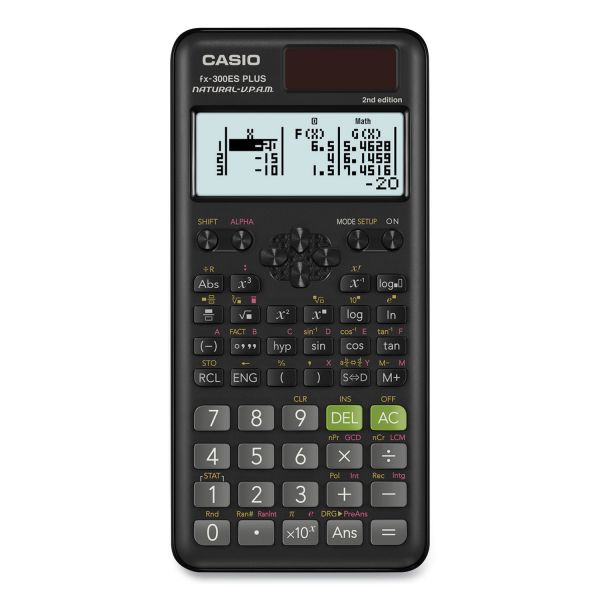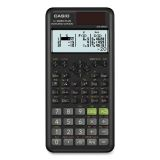# Casio FX-300ES Plus 2nd Edition Scientific Calculator, 16-Digit LCD, Black

Product Number: CSOFX300ESPLS2
• Natural Textbook Display can display fractions, derivatives, integrals, sum and other operations similar to their display in math textbooks.
• Transforms a displayed decimal calculation result to a fraction or mixed number. It can also be converted back to the decimal form.
• Allows you to scroll up on the screen to see and edit previously made calculations.
• 2-function Table of values [f(x), g(x)]: The Table mode allows you to input a function and view the table of values for that function.
More details
\$17.41
Free Shipping Orders Over \$45+ Estimated Delivery Thu 12/9 (3 business days) Thu 12/9 (4 business days)
Favorite
Compare
Request Quote

## Product Description

Casio FX-300ES Plus 2nd Edition Scientific Calculator, 16-Digit LCD, Black - Scientific calculator with sleek new design and slide on hard case. With Natural Textbook Display and improved math functionality. This calculator has been designed as the perfect choice for middle school through high school students learning General Math, Trigonometry, Statistics, Algebra I and II, Pre-Algebra, Geometry, Physics.

• Natural Textbook Display can display fractions, derivatives, integrals, sum and other operations similar to their display in math textbooks.
• Transforms a displayed decimal calculation result to a fraction or mixed number. It can also be converted back to the decimal form.
• Allows you to scroll up on the screen to see and edit previously made calculations.
• 2-function Table of values [f(x), g(x)]: The Table mode allows you to input a function and view the table of values for that function.
• Allows you to determine the set of prime numbers which multiply together to get the original integer.
• Allows the option to divide integers and get an answer as a remainder rather than in decimal form.
• 262 built-in math functions including basic and advanced scientific, exponential and trigonometric, fractions, regression analysis and more.
• Operating system: Entry Logic: V.P.A.M. Greatest Common Divisor, Least Common Multiple.Guides & Information
Related Articles

### Product Specifications

• +/- Switch Key
No
• Amortization
No
• Assembly Required
No
• Backspace Key
Yes
• Base Number Calculations
No
• Bond Calculations
No
• Brand Name
Casio
• Case
Slide-On Hard-Case
• Cash Flow Calculations
No
• Complex Number Calculations
No
• Confidence Interval Calculating
No
• Cost/Sell/Margin
No
• Country of Origin
TH
• Currency Exchange Function
No
• Date Calculations
No
• Decimal Function
Yes
• Depreciation Calculations
No
• Display Angle
Fixed
• Display Characters Height
4 mm
• Display Characters x Display Lines
16 x 4
• Display Notation
Natural Textbook
• Display Type(s)
LCD
• Double Zero Key
No
• Entry Logic
V.P.A.M.
• Equation Editor
Yes
• Fraction/Decimal Conversions
Yes
• Fraction Calculations
Yes
• Global Product Type
Calculators-Scientific
• Grand Total Key
No
• Graphing Functions
No
• Higher Mathematical Functions
Engineering Notation Prime Factorization
• Hyperbolic Functions
Yes
• Hypothesis Testing
Yes
• I/O Port
No
• Interest Rate Conversion
No
• Item Count Function
No
• Linear Regression
Yes
• Loan Calculation
No
• Logical (Boolean) Operations
No
• Markup/Down Key
No
• Matrices
No
• Memory
1 Independent 8 Constant
• Metric Conversion
No
• Number of Display Digits
16
No
• Percent Key(s)
No
• Polar-Rectangular Conversion
No
• Post-Consumer Recycled Content Percent
0%
• Power Source(s)
Battery Solar
• Pre-Consumer Recycled Content Percent
0%
• Probability (Random Number)
Yes
• Recycled
No
• Replacement Batteries
LR44
• Simultaneous Equations
No
• Size
3.15 x 6.37
• Special Features
Black
• Square Root Key
Yes
• Tax Calculation
No
• Time-Value-of-Money
No
• Time/Date
No
• Total Recycled Content Percent
0%
• Trig/Log Functions
Yes
• Variable Regression
Yes
• Variable Statistics
Yes
• Wall-Mountable
No